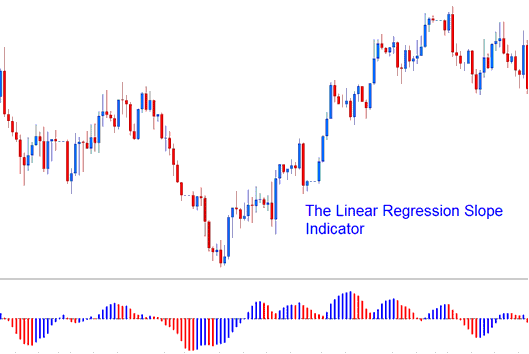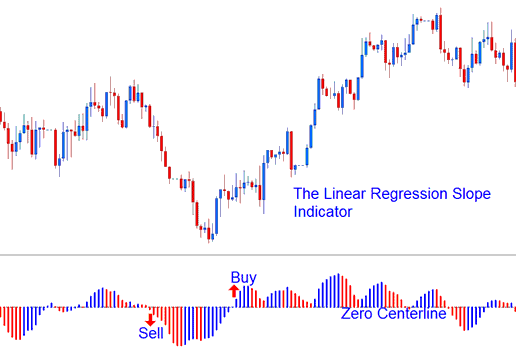# Linear Regression Slope Indices Technical Analysis and Linear Regression Slope MT5 Stock Indexes Indicator

The Linear Regression Slope calculates the slope/gradient value of regression lines which involve the current stock indexes price bar and the previous n-1 stock indexes price bar (where n = regression periods)

This MetaTrader 5 Indices Indicator calculates this value and updates it for each stock indexes price candlestick loaded in the stock indexes price chart.

The Indicator is calculated from the Linear Regression Indices Technical Indicator. The linear regression draws the indices trend of the stock indexes price chart over a specified duration of time and this market indices trend is determined by plotting a Linear Regression Indices Trend Line using the "least squares fit" method. The slope of this indices trend line is then calculated and this forms the linear regression.Linear Regression Slope

The slope values are then smoothed by multiplying the raw slope indicator values by 100 and then dividing this value by the indices price

Linear Slope Regression = (raw value of slope * 100 / indices price).

The smoothing of the slope values is essential when comparing markets that are volatile and trade within wide stock indexes price ranges for each stock indexes price candlestick. The smoothed slope value will show the percent change in the stock indexes price per every candlestick used to calculate the regression (best fit) line.

## Indices Technical Analysis and Generating Indices Trading Signals

• If the smoothing of the slope is 0.30, then the regression line is rising and adjusting at a rate of 0.30% for every candlestick.

• If the smoothing of the slope of -0.30, then the regression line is going down and adjusting at a rate of -0.30% for every candlestick.

The regression slope is displayed as a bi-color histogram that oscillates above and below zero center line. The center line that is used to generate indices trading signals is set at the 0 level.

• A rising slope (greater than the previous value of 1 candlestick ago) is displayed in the Blue/Upward Slope color,

• A declining slope (lower than the previous value of 1 candlestick ago) is displayed in the Red/Downward Slope color.Technical Analysis